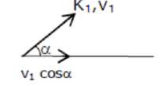# An electron with kinetic energy

Question:

An electron with kinetic energy $K_{1}$ enters between parallel plates of a capacitor at an angle ' $\alpha$ ' with the plates, It leaves the plates at angle ' $\beta^{\prime}$ with kinetic energy $K_{2}$. Then the ratio of kinetic energies $K_{1}: K_{2}$ will be:

1. (1) $\frac{\sin ^{2} \beta}{\cos ^{2} \alpha}$

2. (2) $\frac{\cos ^{2} \beta}{\cos ^{2} \alpha}$

3. (3) $\frac{\cos \beta}{\sin \alpha}$

4. (4) $\frac{\cos \beta}{\cos \alpha}$

Correct Option: , 2

Solution:

(2)$\because v_{1} \cos \alpha=v_{2} \cos \beta$

$\frac{v_{1}}{v_{2}}=\frac{\cos \beta}{\cos \alpha}$

Then the ratio of kinetic energies

$\frac{k_{1}}{k_{2}}=\frac{\frac{1}{2} m v_{1}^{2}}{\frac{1}{2} m v_{2}^{2}}=\left(\frac{v_{1}}{v_{2}}\right)^{2}=\left(\frac{\cos \beta}{\cos \alpha}\right)^{2}$

$\frac{k_{1}}{k_{2}}=\frac{\cos ^{2} \beta}{\cos ^{2} \alpha}$